## Count number of dates by year, month in Excel

When working on Excel worksheet, sometimes, you may want to count the cells where dates from a given year or month as below screenshot shown. To solve this task in Excel, you can use the SUMPRODUCT, YEAR and MONTH functions to create formulas to count the number of dates belongs to the specific year or month as you need.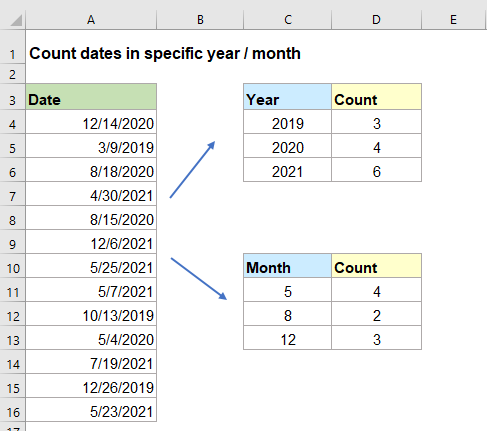#### Count number of dates of given year

To count the number of dates in a given year, you can combine the SUMPRODUCT and YEAR functions together, the generic syntax is:

=SUMPRODUCT(--(YEAR(date_range)=year))
• date_range: A list of cells contain the dates you want to count;
• year: The value or cell reference that represents the year you want to count for.

1. Enter or copy the below formula into a blank cell where you want to get the result:

=SUMPRODUCT(--(YEAR(\$A\$2:\$A\$14)=C2))

Note: In this formula, A2:A14 is the range of cells contain the dates, C2 contain the specific year you want to count for.

2. Then, drag the fill handle down to apply this formula to other cells, and you will get the number of dates based on the given year, see screenshot: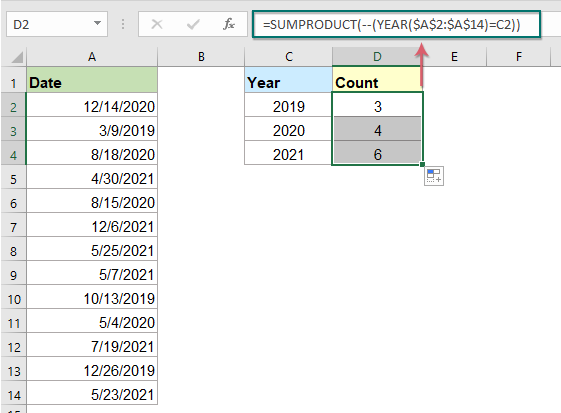##### Explanation of the formula:

=SUMPRODUCT(--(YEAR(\$A\$2:\$A\$14)=C2))

• YEAR(\$A\$2:\$A\$14)=C2: The YEAR function extracts the year value from the list of dates as this: {2020;2019;2020;2021;2020;2021;2021;2021;2019;2020;2021;2019;2021};
And then, each year is compared to the year value in cell C2, to return an array of TRUE and FALSE values: {FALSE;TRUE;FALSE;FALSE;FALSE;FALSE;FALSE;FALSE;TRUE;FALSE;FALSE;TRUE;FALSE}.
• --(YEAR(\$A\$2:\$A\$14)=C2)=--{FALSE;TRUE;FALSE;FALSE;FALSE;FALSE;FALSE;FALSE;TRUE;FALSE;FALSE;TRUE;FALSE}: --this double negative sign converts TRUE value to 1 and False value to 0. So, you will get the result as this: {0;1;0;0;0;0;0;0;1;0;0;1;0}.
• SUMPRODUCT(--(YEAR(\$A\$2:\$A\$14)=C2))= SUMPRODUCT({0;1;0;0;0;0;0;0;1;0;0;1;0}): Finally, this SUMPRODUCT function sums all the items in the array, and return the result: 3.

#### Count number of dates of given month

If you want to count the number of dates based on a given month, the SUMPRODUCT and MONTH functions may do you a favor, the generic syntax is:

=SUMPRODUCT(--(MONTH(date_range)=month))
• date_range: A list of cells contain the dates you want to count;
• month: The value or cell reference that represents the month you want to count for.

1. Enter or copy the below formula into a blank cell where you want to get the result:

=SUMPRODUCT(--(MONTH(\$A\$2:\$A\$14)=C2))

Note: In this formula, A2:A14 is the range of cells contain the dates, C2 contain the specific month you want to count for.

2. Then, drag the fill handle down to apply this formula to other cells, and you will get the number of dates based on the given month, see screenshot: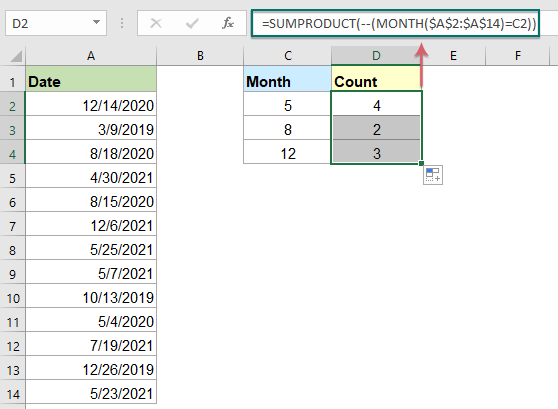##### Explanation of the formula:

=SUMPRODUCT(--(MONTH(\$A\$2:\$A\$14)=C2))

• MONTH(\$A\$2:\$A\$14)=C2: This MONTH function extracts the month number from the list of dates as this: {12;3;8;4;8;12;5;5;10;5;7;12;5}.
And then, each month is compared to the month number in cell C2, to return an array of TRUE and FALSE values: {FALSE;FALSE;FALSE;FALSE;FALSE;FALSE;TRUE;TRUE;FALSE;TRUE;FALSE;FALSE;TRUE}.
• --(MONTH(\$A\$2:\$A\$14)=C2)= --{FALSE;FALSE;FALSE;FALSE;FALSE;FALSE;TRUE;TRUE;FALSE;TRUE;FALSE;FALSE;TRUE} : - -this double negative sign converts TRUE value to 1 and False value to 0. So, you will get the result as this: {0;0;0;0;0;0;1;1;0;1;0;0;1}.
• SUMPRODUCT(--(MONTH(\$A\$2:\$A\$14)=C2))= SUMPRODUCT({0;0;0;0;0;0;1;1;0;1;0;0;1}): This SUMPRODUCT function sums all the items in the array, and return the result: 4.

#### Count number of dates by both year and month

For counting the number of dates based on both year and month, for example, I want to know how many dates are there in May 2021.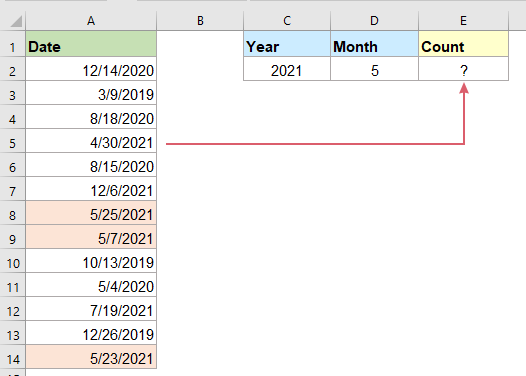In this case, you can use a combination of SUMPRODUCT, MONTH and YEAR functions to get the result, the generic syntax is:

=SUMPRODUCT((MONTH(date_range)=month)*(YEAR(date_range)=year))
• date_range: A list of cells contain the dates you want to count;
• month: The value or cell reference that represents the month you want to count for;
• year: The value or cell reference that represents the year you want to count for.

Please enter or copy the below formula into a blank cell to output the result, and then press Enter key to get the calculation, see screenshot:

=SUMPRODUCT((MONTH(\$A\$2:\$A\$14)=D2)*(YEAR(\$A\$2:\$A\$14)=C2))

Note: In the above formula, A2:A14 is the range of cells contain the dates, D2 contain the specific month and C2 is the year number that you want to count for.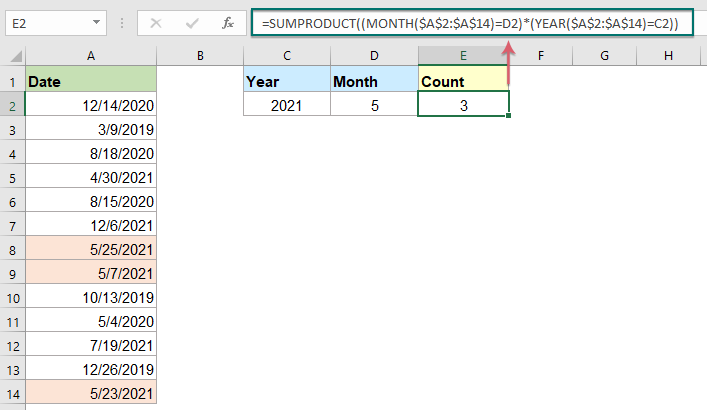#### Relative function used:

• SUMPRODUCT:
• The SUMPRODUCT function can be used to multiply two or more columns or arrays together, and then get the sum of products.
• MONTH:
• The Excel MONTH function extracts the month from a date and displays as integer number from 1 to 12.
• YEAR:
• The YEAR function returns the year based on the given date in a 4-digit serial number format.

#### More articles:

• Count Number Of Cells Between Two Values / Dates
• Have you ever tried to get or count the number of cells between two given numbers or dates in Excel as below screenshot shown? This article will talk about some useful formulas for dealing with it.
• Count Number Of Date Cells In A Range By Day Of Week
• Excel provides the combination of SUMPRODUCT and WEEKDAY functions to help us easily count the number of specified weekdays within a range. This tutorial provides a step by step guide to help you get through it.
• Count Number Of Cells That Contain Specific Text
• Supposing, you have a list of text strings, and may want to find the number of cells that contain certain text as part of their contents. In this case, you can use the wildcard characters (*) that represents any texts or characters in your criteria when applying the COUNTIF function. This article, I will introduce how to use formulas for dealing with this job in Excel.

### The Best Office Productivity Tools

#### Kutools for Excel - Helps You To Stand Out From Crowd

Would you like to complete your daily work quickly and perfectly? Kutools for Excel brings 300 powerful advanced features (Combine workbooks, sum by color, split cell contents, convert date, and so on...) and save 80% time for you.

• Designed for 1500 work scenarios, helps you solve 80% Excel problems.
• Reduce thousands of keyboard and mouse clicks every day, relieve your tired eyes and hands.
• Become an Excel expert in 3 minutes. No longer need to remember any painful formulas and VBA codes.
• 30-day unlimited free trial. 60-day money back guarantee. Free upgrade and support for 2 years.#### Office Tab - Enable Tabbed Reading and Editing in Microsoft Office (include Excel)

• One second to switch between dozens of open documents!
• Reduce hundreds of mouse clicks for you every day, say goodbye to mouse hand.
• Increases your productivity by 50% when viewing and editing multiple documents.
• Brings Efficient Tabs to Office (include Excel), Just Like Chrome, Firefox, And New Internet Explorer.